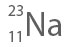## Calculating sub-atomic particles from atomic number and mass number

We can calculate the number of sub-atomic particles (i.e. electrons, protons, neutrons) if the atomic mass or atomic number is provided for an element. Similarly, the atomic number and mass number can be calculated for any element if the number of subatomic particles is known.

From the definitions of Atomic number and Atomic mass, we know:

Atomic number  =  the number of protons

Mass number    =  the number of protons + the number of neutrons

From these, we can deduct:

Number of neutrons = Mass number – Atomic number

Atom has no overall charge, which means the there are equal number of negatively charged electrons and positively charged protons. If we know the number of protons (or atomic number) of an atom, this will be equal to the number of electrons of that atom.

Number of electrons = Number of protons

## Example Question:

Calculate the sub-atomic particles for## Solution:

From the provided data, we know:

Mass number: 23
Atomic number: 11

As we know:
Number of protons = Atomic number

Therefore,
Number of protons = 11

Since, Number of electrons = Number of protons
Therefore, Number of electrons = 11

As we know:

Mass number = number of protons + neutrons
and, Atomic number = number of protons

Therefore,

Number of neutrons = Mass number – Atomic number

= 23 – 11

Number of neutrons = 12